Home » GRE » GRE Quant » GRE Quant Formulas - Cheat sheet for GRE Preparation

# GRE Quant Formulas - Cheat sheet for GRE Preparation

•#### What are some of the basic GRE® Quant formulas? Below you will find some of the most important and basic math formulas that will be helpful in your GRE® quantitative section. They can also be called GRE® Quant formulas or GRE® math formulas. ### 1. Square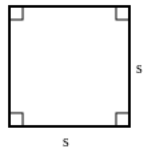PerimeterP = 4SMultiply any one side (s) by four.AreaA = (side)^2Multiply any two sides together (i.e., square one side). ### 2. Rectangle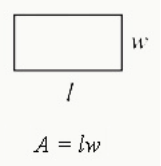PerimeterP = 2(l+w)Multiply the length (l) by 2 and the width (w) by 2, and then add the products together.AreaA = l*wMultiply the length by the width. ### 3. Circle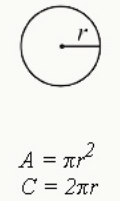PerimeterP = 2πr or πdMultiply 2, π (pi), and the radius (r) (the length of a line connecting the center of the circle to the edge).Alternatively, multiply π by the diameter (d) (the length of a line cutting the circle in half).Two radii (the plural of radius) equal the diameter, so 2r=d.π can be rounded to 3.14 (or 3.14159).AreaA =πr^2Square the radius and multiply it by π.Note: all circles equal 360 degrees. ### 4. Triangle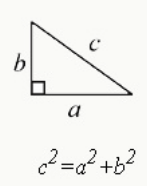Pythagorean Theorem(a)^2 + (b)^2 = c^2This theorem can only be used for right triangles (triangles with a 90-degree angle).a and b are the two shorter sides, or “legs,” and c is the hypotenuse (the longest side of a right triangle).Certain triangle-side combinations (a:b:c), called Pythagorean triples, are easy to memorize. Common ones you may come across on the GRE® are:3:4:55:12:138:15:17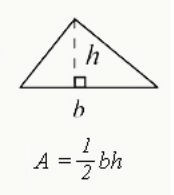AreaA =1/2 bhMultiply the base (b) by the height (h) and divide by 2.Note: angles in a triangle always add up to 180 degrees. ### 5. Trapezoid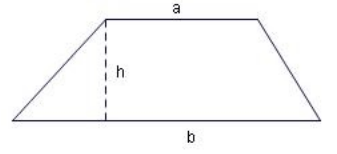AreaA = a+b2ha and b are parallel sides.Add a and b, divide by 2, and then multiply by the height (h). ### 6. Laws of Exponents x 0 = 1x -1 =1x, x-2 =1x2, etcxa xb = xa + bx axb = x(a-b)=1xb-axaya=(xy)a(xy)a=xaya(xa)b=xab ### 7. Laws of Square Roots a square =aab=abab=ab ### 8. Laws of Even and Odd Numbers even + even = evenodd + odd = eveneven + odd = oddeven * even = evenodd * odd = oddeven * odd = even ### 9. Distance D =rtMultiply the rate (r) by the time (t) to find the distance (D).You can also solve for the time or rate by rearranging this formula to equal either r or t: R =Dt or t=Dr ### 10. Slope of a Line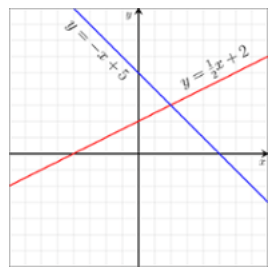Using the Equation of a Liney=mx+bA slope is the steepness of a line in a coordinate system.m is the slope. x and y are a pair of coordinates.b is the y-intercept, or where the line passes through the y-axis.You may occasionally see this equation written in a different way. Always convert it to the format above to ease calculations and avoid confusion.A line increasing as it moves left to right has a positive slope, whereas a decreasing line has a negative slope. A completely horizontal line has a slope of 0.If the y-intercept of a line is 0, the formula for it is y=mx+0 or y=mx. Here is an example: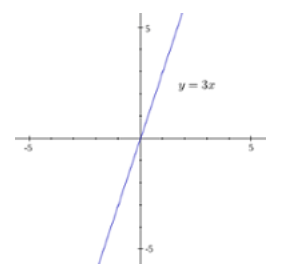Using Two Sets of Coordinatesm = (y2 – y1)/(x2- x1)orslope=rise/runx1 and y1 are a corresponding pair of coordinates on a line. (x2 and y2 are a separate pair of coordinates on the same line).This equation is known as rise over run (the change in vertical distance over the change in horizontal distance). ### 11. Average average = sum of n numbers/naverage speed = total distance/total timeThe average is also called the mean.Don’t confuse the average for other statistical terms. Common terms you may see on the GRE® test are:Mode: the most common number of a data setRange: the difference between the highest number and the lowest number of a data set.Median: the middle number of a data set.### 12. Probability Probability of an event occurring = number of successful outcomes/ total number of possible outcomes.Probability of two independent events occurring = probability of event A * probability of event BProbabilities are usually written as fractions, though you may see them written as decimals or ratios (e.g., 3:4).### 13. Percentages Percent BasicsSolve for x percent of number nN x 100Alternatively, a faster way to solve this is by moving the decimal point of the percent to the left two places and multiplying it by n. For example, what is 12 percent of 50? Answer: 50*(12/100) = 6.Solve for what number n is x percent of:100 nxSolve for what percent is number n of number m:100 nmPercent ChangePercent IncreaseFinal amount – original amount/original amount *100.The numerator is equivalent to the actual increase in the amount.Percent Decrease((Original amount –final amount)/(original amount)) *100The numerator is equivalent to the actual decrease in the amount.Do you need to memorize GRE® Quant formulas?While the GRE® is not a test of knowledge, you will need to show up on the test day with some basic math formula memorized. Though memorizing Quant formulas isn't the only way to study for the GRE® Quant, memorizing certain frequently-tested math formulas will help you improve your speed on test day. Once you learn these GRE® math formulas, be sure to practice using them! Timed practice will ensure you can answer all the questions giving you a higher chance to ace your test.Time allocated for the GRE® Quant section and knowing your GRE® Quant formulas.The Quant section has 40 questions in total which are divided into 2 sections each containing 20 questions and the time allocated for each part is 35 minutes. In total, the GRE® Quant sections take 1 hour 10 minutes. Therefore, knowing your quant formulas and keeping a handy math formula chart for your practice sessions is key.GRE Score : 330 ( V : 160 | Q : 170)
Each and every topic is covered in the program. I did not refer to any other books apart from the material provided by quant and verbal guides and the official guide. They give us feedback after every test and tell us our where we need to improve.Apoorva Saxena
GRE Score : 329 ( V : 159 | Q : 170)
GREedge was my first experience of online coaching. And due to their very methodical approach, it was great one. They were thorough, they were very much involved and they monitored my performance minutely. It actually proved the best of both worlds.Divya Murali
GRE Score : 334 ( V : 164 | Q : 170)
I took the GREedge one month online course and it was of great use to me. It has lessons on each topic and also practice sessions. At the end there is also full length tests available for practice. Nothing beats Wordbot for vocab building!Priyanka Sundaram
GRE Score : 307 ( V : 149 | Q : 158)
I was inspired and motivated throughout the program and this helped me to reduce the tension at the last moment. I owe my success entirely to GREEdge. They made me realise my true potential and I am always grateful for it. Thank you GREedge!Rishikesh Mishra
GRE Score : 309 ( V : 149 | Q : 160)
I think GREedge is the best. I have scored 309 marks in GRE with the help of GREedge.I took the one-month study plan of GREedge and it proved to be very useful. GREedge provides you a perfect interface for learning and then testing your skills.Chirag Venkateshan
GRE Score : 310 ( V : 150 | Q : 160)
This two-month long program was worth every penny because of the Personal SFAs assigned to me, the detailed schedule, WordBot, and exhaustive analysis of my performance as compared previously successful student. That was a game changer!Pulkit Verma
GRE Score : 325 ( V : 155 | Q : 170)
What I really liked about GRE Edge was their SFAs (Student Facilitator and Analysts). Every student is assigned two SFAs when they enroll for the program and they help in keeping track of your progress during the preparations.Uruba Andaleeb
GRE Score : 307 ( V : 150 | Q : 157)
GREedge made GRE way simpler! With so many materials available to prepare for GRE, it was so confusing for me. But GREedge gave me a proper direction, and it was an amazing experience. I would recommend this program to any GRE aspirant.Rahul Reddy
GRE Score : 320 ( V : 156 | Q : 164)
Opening the GREedge learning tracker excited me every day. It's because it is designed in such a way that it engages students in it and urges them to complete their tasks daily and regularly. It was systematic and personalized. Good work design team :)Abishek Surya
GRE Score : 310 ( V : 155 | Q : 155)
I never felt alone with GREedge. It was fascinating to see my trainers be as passionate about my ambitions and goals as much as I am! It’s like they’ve got your back, and they want to make it happen for you. I did not have to feel stressed about it.
##### Tags